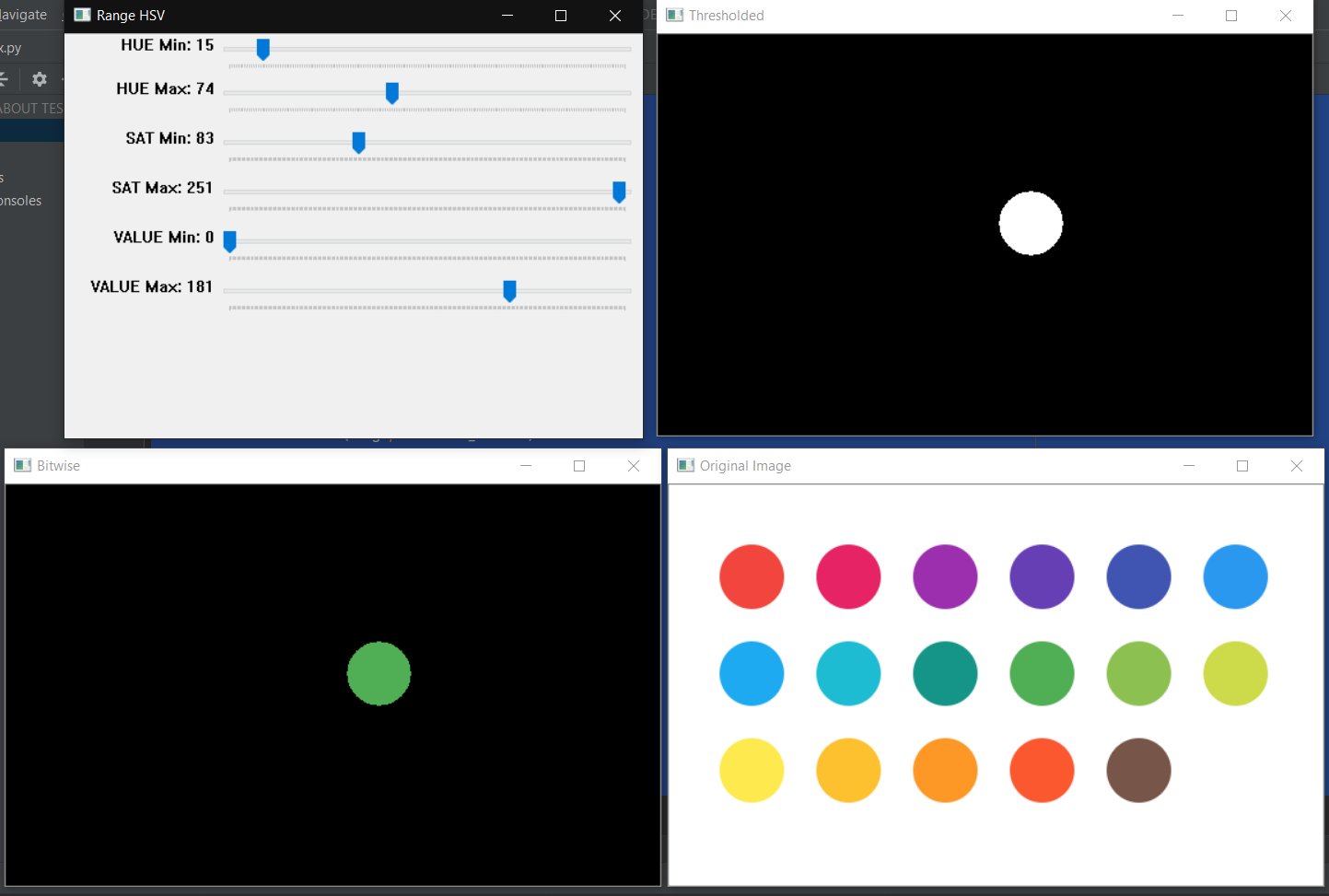Hello friends, in this tutorial I will create a programming program using OpenCV colors using the Python programming language.

So, for those who don't know OpenCV is an open-source library that can be used for real-time dynamic image processing, created by Intel, and now supported by Willow Garage and Itseez. I made this program using the PyCharm JetBrains IDE.

First, open your PyCharm and then create a new file, for example I use the name "index.py" and add an image that has a variety of colors like thiscolors.png
If you have typed the following code in index.py

```import cv2
import numpy as np

def empty(a):
pass

# create new window with trackbar for HSV Color

cv2.namedWindow("Range HSV")
cv2.resizeWindow("Range HSV", 500, 350)
cv2.createTrackbar("HUE Min", "Range HSV", 0,180,empty)
cv2.createTrackbar("HUE Max", "Range HSV", 180,180,empty)
cv2.createTrackbar("SAT Min", "Range HSV", 0,255,empty)
cv2.createTrackbar("SAT Max", "Range HSV", 255,255,empty)
cv2.createTrackbar("VALUE Min", "Range HSV", 0,255,empty)
cv2.createTrackbar("VALUE Max", "Range HSV", 255,255,empty)

```

In the above code, we enter the required libraries namely cv2 and numpy, then we create an empty function. Then we create a new window with the name HSV Range in which will contain 6 trackbar and the last we create a variable with the name of the image to read the image to be inserted.

If we have entered the next code

```while True:

# get value from trackbar
h_min = cv2.getTrackbarPos("HUE Min", "Range HSV")
h_max = cv2.getTrackbarPos("HUE Max", "Range HSV")
s_min = cv2.getTrackbarPos("SAT Min", "Range HSV")
s_max = cv2.getTrackbarPos("SAT Max", "Range HSV")
v_min = cv2.getTrackbarPos("VALUE Min", "Range HSV")
v_max = cv2.getTrackbarPos("VALUE Max", "Range HSV")

# define range of some color in HSV

lower_range = np.array([h_min,s_min,v_min])
upper_range = np.array([h_max, s_max, v_max])

# convert image to HSV

hsv = cv2.cvtColor(image, cv2.COLOR_BGR2HSV)

# threshold the hsv image to get some color

thresh = cv2.inRange(hsv, lower_range, upper_range)

# bitwise AND mask and original image

cv2.imshow("Original Image", image)
cv2.imshow("Thresholded", thresh)
cv2.imshow("Bitwise", bitwise)

k = cv2.waitKey(1) & 0xFF
if k == ord('m'):
mode = not mode
elif k == 27:
break
cv2.destroyAllWindows()
```

The code above is a looping continuously, inside there are several variables, i.e.

• h_min : to store numeric values ​​from the trackbar with the name HUE Min
• h_max : to store numeric values ​​from the trackbar with the name HUE Max
• s_min : to store numeric values ​​from the trackbar with the name SAT Min
• s_max : to store numeric values ​​from the trackbar with the name SAT Max
• v_min : to store numeric values ​​from the trackbar with the name VALUE Min
• v_max : to store numeric values ​​from the trackbar with the name VALUE Max

Next, there are lower_range and upper_range variables, where lower_range will store the values ​​of HUE Min, SAT Min and VALUE Min in the form of arrays. Where as upper_range is to store HUE Max, SAT Max and VALUE Max values ​​in an array.

Next we convert the image variable into HSV mode which is stored in the hsv variable. Furthermore, the hsv variable was masked or threshold based on the range of values ​​of the lower_range and upper_range variables. Finally in the bitwise variable we display the detected color.

If it's finished, we display these variables, namely image, thresh and bitwise. The code snippet below works so that the form doesn't close immediately, meaning that if we want to close it we need to press the "m" button on the keyboard.Results